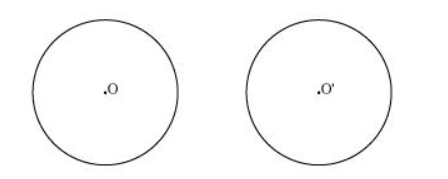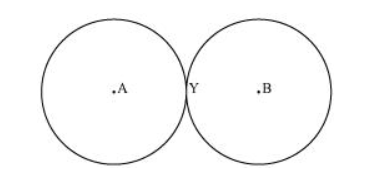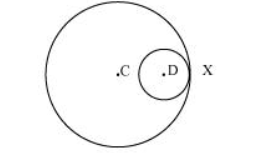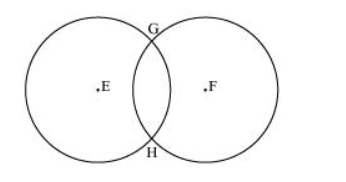# Draw different pairs of circles. How many points does each pair have in common?

Question. Draw different pairs of circles. How many points does each pair have in common? What is the maximum number of common points?

Solution:

Consider the following pair of circles.The above circles do not intersect each other at any point. Therefore, they do not have any point in common.The above circles touch each other only at one point Y. Therefore, there is 1 point in common.The above circles touch each other at 1 point X only. Therefore, the circles have 1 point in common.These circles intersect each other at two points G and H. Therefore, the circles have two points in common. It can be observed that there can be a maximum of 2 points in common. Consider the situation in which two congruent circles are superimposed on each other. This situation can be referred to as if we are drawing the circle two times.